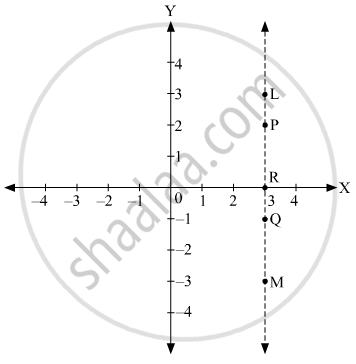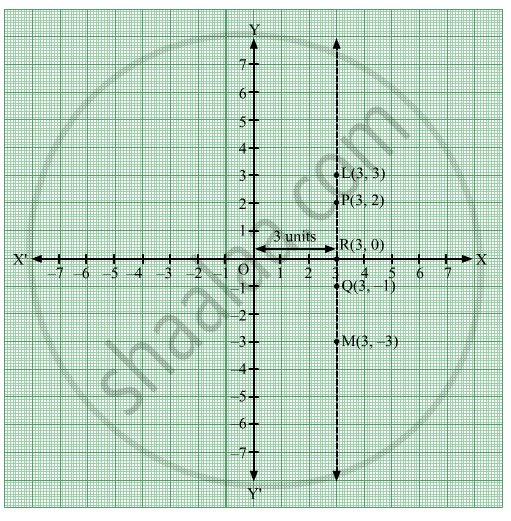Advertisement Remove all ads

# In the Graph Alongside, Line Lm is Parallel to the Y-axis. - Geometry

Graph

In the graph alongside, line LM is parallel to the Y-axis.1.  What is the distance of line LM from the Y-axis ?

2.  Write the co-ordinates of the points P, Q and R.

3.  What is the difference between the x co-ordinates of the points L and M?
Advertisement Remove all ads

#### Solution1. The line LM is parallel to the Y-axis and passing through the point (3, 0).

The equation of the line LM is x = 3 and at a distance of 3 units to the right of the Y-axis.

Thus, the distance of line LM from the Y-axis is 3 units.

2. The x co-ordinate of a point is its distance from the Y-axis and y co-ordinate is its distance from the X-axis.

Thus, the co-ordinates of the points P, Q and R are (3, 2), (3, −1) and (3, 0), respectively.

3.
x co-ordinate of point L = 3

x co-ordinate of point M = 3

∴ Difference between the x co-ordinates of the points L and M = 3 − 3 = 0

Thus, the difference between the x co-ordinates of the points L and M is 0.

Is there an error in this question or solution?
Advertisement Remove all ads

#### APPEARS IN

Balbharati Mathematics 2 Geometry 9th Standard Maharashtra State Board
Chapter 7 Co-ordinate Geometry
Problem Set 7 | Q 5 | Page 99
Advertisement Remove all ads
Advertisement Remove all ads
Share
Notifications

View all notifications

Forgot password?